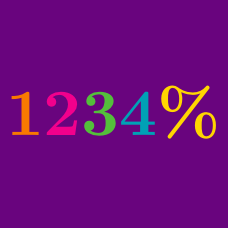Everyday Math

# Converting Percentages and Fractions

Which of the following is equal to $\frac{ 1} { 5 }$?

Which of the following is equal to $20\%$ of $\frac{ 1} { 5 }?$

In the diagram below, find the product of the proportion of addition signs and the proportion of subtraction signs to all the arithmetic signs.Details and assumptions:

• Each sign is counted as one regardless of its size or kind.

Which of the following is equal to $\dfrac{3}{8} \times \dfrac{5}{6}$?

Which of the following is equal to $5 \%$?

×